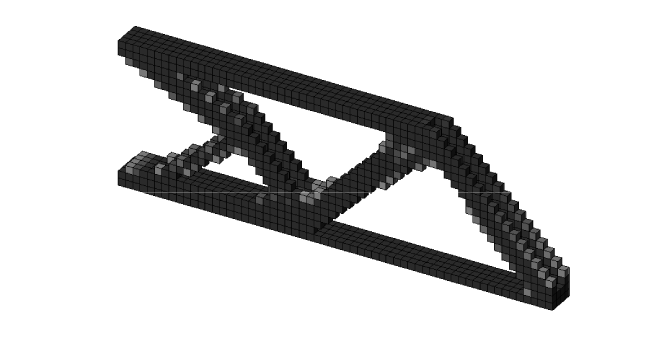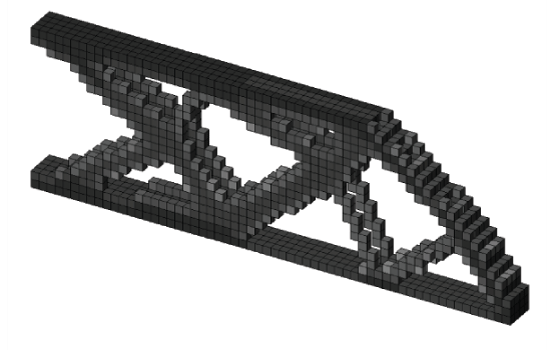# [Filters] Grayscale Filter - Top3d

In the topology optimization, filters are introduced to avoid numerical instabilities. Different filtering techniques may result different discreteness of the final solutions, and sometimes may even contribute to different topologies. In this series of tutorial, you will learn how to implement sensitivity filter and gray scale filter in the top3d program.

In the topology optimization, filters are introduced to avoid numerical instabilities. Different filtering techniques may result different discreteness of the final solutions, and sometimes may even contribute to different topologies. In addition to density filter, in the literatures there are bunch of different filtering schemes. For example, sensitivity filter, morphology based black and white filters, filtering technique using Matlab built-in function conv2, filtering based on Helmholtz type differential equations, Heaviside filter, and gray scale filter. All the filters pursue a simple goal to achieve black-and-white structures. Two of them are chosen, which stand for classic and better performance, as well as easy implementation.

A simple non-linear grayscale filter or intermediate density filter has been proposed by Groenwold to further achieve black-and-white topologies. The implementation of the grayscale filter is by changing the OC update scheme as the following

The standard OC updating method is a special case of equation above with $q=1$. A typical value of $q$ for the SIMP-based topology optimization is $q=2$.

The implementation of the grayscale filter to the code can be done as follows:

### Step.1: Adding One Input Variable

Change line 2 by adding one input variable $q$ to the program

function top3dgsf(nelx,nely,nelz,volfrac,penal,qmax,rmin)


### Step.2: Changing OC subroutine

Changing line 85 as follows

xnew = max(0,max(x-move,min(1,min(x+move,(x.*sqrt(-dc./dv/lmid)).^q))));


### Step.3: Continuations

The factor $q$ should be increased gradually by adding one line after line 68

if loop <= 15, q = 1; else q = min(qmax,1.01*q); end


### Step.4: Results

The default cantilever beam program solved using grayscale filter is shown below (left) compared with those using density filter (right)